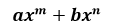# Binomial

Binomial is a polynomial having only two terms in it. The equation formed is an algebraic expression. In Maths you will come across many topics related to this concept.  Here we will learn its definition, examples, formulas, operations performed on equations, such as addition, subtraction, multiplication and about Binomial expansion.

## Binomial Definition

The algebraic expression which contains only two terms is called binomial. It is a two-term polynomial. Also, it is called as a sum or difference between two or more monomials. It is the simplest form of a polynomial.

• When expressed as a single indeterminate, a binomial can be expressed as;Where a and b are the numbers, and m and n are non-negative distinct integers. x takes the form of indeterminate or a variable.

• In Laurent polynomials, binomials are expressed in tha same manner, but the only difference is m and n can be negative. Therefore, we can write it as;

ax-m + bx-n

### Examples

Some of the examples are;

• 4x2+5y2
• xy2+xy
• 0.75x+10y2

## Operations on Binomials

There are few basic operations that can be carried out on this two-term polynomials are.

#### Factorization

We can factorise and express a binomial as a product of the other two.
For example, x– ycan be expressed as (x+y)(x-y).

Addition of two binomials is done only when it contains like terms. This means that it should have the same variable and the same exponent.
For example,
Let us consider, two equations.
10x3 + 4y and 9x3 + 6y
Adding both the equation = (10x3 + 4y) + (9x3 + 6y)
Therefore, the resultant equation = 19x3 + 10y

#### Subtraction

Subtraction of two binomials is similar to the addition operation as if and only if it contains like terms.
For example,
12x3 + 4y and 9x3 + 10y
Subtracting the above polynomials, we get;

(12x3 + 4y) – (9x3 + 10y)
= 12x3 + 4y – 9x3 – 10y
Therefore, the resultant equation is = 3x3 – 6y

#### Multiplication

When multiplying two binomials, the distributive property is used and it ends up with four terms. It is generally referred to as the FOIL method. Because in this method multiplication is carried out by multiplying each term of the first factor to the second factor. So, in the end, multiplication of two two-term polynomials is expressed as a trinomial.

For example, (mx+n)(ax+b) can be expressed as max2+(mb+an)x+nb

#### Raising to nth Power

A binomial can be raised to the nth power and expressed in the form of;

(x + y)n

#### Converting to lower order binomials

Any higher order binomials can be factored down to lower order binomials such as cubes can be factored down to products of squares and another monomial.

For example, x+ y3 can be expressed as (x+y)(x2-xy+y2)

## Binomial Expansion

In Algebra, binomial theorem defines the algebraic expansion of the term (x + y)n. It defines power in the form of axbyc. The exponents b and c are non-negative distinct integers and b+c = n and the coefficient ‘a’ of each term is a positive integer and the value depends on ‘n’ and ‘b’.

For example, for n=4, the expansion (x + y)4 can be expressed as

(x + y)4 = x4 + 4x3y + 6x2y+ 4xy3 + y4

The coefficients of the binomials in this expansion 1,4,6,4, and 1 forms the 5th degree of Pascal’s triangle.

The general theorem for the expansion of (x + y)n is given as;

(x + y)n = ${n \choose 0}x^{n}y^{0}$+${n \choose 1}x^{n-1}y^{1}$+${n \choose 2}x^{n-2}y^{2}$+$\cdots$+${n \choose n-1}x^{1}y^{n-1}$+${n \choose n}x^{0}y^{n}$

Some of the methods used for the expansion of binomials are :

• Pascal’s Triangle
• Factorials
• Combinations
• Binomial Theorems
• Binomial Series

### Binomial Equation

Any equation that contains one or more binomial is known as a binomial equation.

Some of the examples of this equation are:

x+ 2xy + y= 0

v = u+ 1/2 at2

### Binomial Example

Question: Find the binomial from the following terms?

1. 5x + 6y
2. 5 * y
3. 6xy
4. 6x ÷ y

Solution:

1. Option 1 : 5x + 6y : Here, addition operation makes the two terms from the polynomial
2. Option 2 : 5 * y : Multiplication operation produces 5y as a single term
3. Option 3 : 6xy : Multiplication operation produces the polynomial 6xy as a single term
4. Option 4 : 6x÷ y : Division operation makes the polynomial as a single term.

Therefore, the solution is 5x + 6y, is a binomial that has two terms.

Learn more about binomials and related topics in a simple way. Register with BYJU’S – The Learning App today.